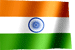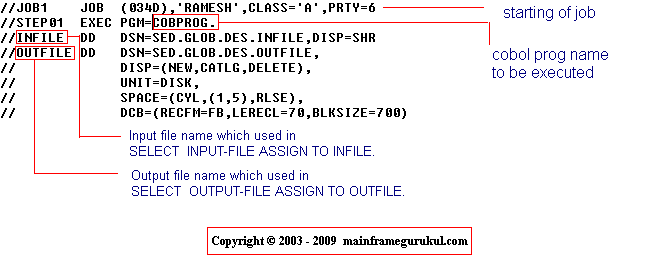RAMESH KRISHNA REDDYOnline Tutorials | PREV | TOP | NEXT

DRONA SERIES
JCL STUDY MATERIAL

CHAPTER - 1

 ``` INTRODUCTION Job control language. It is a means of communication between a program that can be written in COBOL , ASSEMBER or PL/I and the MVS operating system. Without a JCL, you cant able to run a job on MVS operating system. Let us start with an example jcl, how it looks like, The following jcl is used to run an cobol program. I will explain in this chapter each and every line of this jcl. If you are already fimiliar with jcl, please skip first 6 chapters. (First 1 - 6 chapters intended for beginners) JCL , used to run a cobol program //JOB1 JOB (034D),'RAMESH KRISHNA REDDY',CLASS='A',PRTY=6 //STEP01 EXEC PGM=COBPROG. //INFILE DD DSN=SED.GLOB.DES.INFILE,DISP=SHR //OUTIFLE DD DSN=SED.GLOB.DES.OUTFILE, // DISP=(NEW,CATLG,DELETE), // UNIT=DISK, // SPACE=(CYL,(1,5),RLSE), // DCB=(RECFM=FB,LERECL=70,BLKSIZE=700) to see source code of cobol program COBPROG click here Now let us discuss JCL which we have seen. Before explaining, I will explain few basics about JCL All JCL statements can consists of up to five types of fields // The two forward slashes are required at the beginning of each JCL statement in Columns 1 and 2. Name field - This is an optional field. If coded, should start at Column 3. It maximum length is 8. Operation field - Which indicates the operation that is to be performed Operand - This field must appear after Operation field. Must start at 16 column Comments - Comments begin one space after the last operand. Our simple example has no comments. // Name Operation OPerand // JOB1 JOB (034D), 'RAMESH', CLASS='A', PRTY=6 // STEP01 EXEC PGM=COBPROG // INFILE DD DSN=SED.GLOB.DES.INFILE, DISP=SHR // OUTIFLE DD DSN=SED.GLOB.DES.OUTFILE, // DISP=(NEW,CATLG,DELETE), // UNIT=DISK, // SPACE=(CYL,(1,5),RLSE), // DCB=(RECFM=FB, // LERECL=70,BLKSIZE=700) The following image explain How our JCL will execute cobol programEXPLANATION - //JOB1 JOB (034D),'RAMESH',CLASS='A',PRTY=6 job card is mainly used to identify job, and tell to MVS about type of job it is, priority it has. JOB1 is the job name 034D is accounting information RAMESH is name of the programmer CLASS is category of job PRTY is priority in the class //STEP01 EXEC PGM=COBPROG EXEC is used to specify the program to be executed. STEP01 is the name of the step (you can give any name here) COBPROG is the program name to be executed //INFILE DD DSN=SED.GLOB.DES.INFILE,DISP=SHR INFILE is the name of input file, which is used in cobol program DSN=SED.GLOB.DES.INFILE - It is dataset contains actual information to be read. DISP=SHR - means another program also can read this program while we are reading this file. //OUTFILE DD DSN=SED.GLOB.DES.OUTFILE, // DISP=(NEW,CATLG,DELETE), // UNIT=DISK, // SPACE=(CYL,(1,5),RLSE), // DCB=(RECFM=FB,LERECL=70,BLKSIZE=700) OUTFILE is the name of outputfile, which is used in cobol program DSN=SED.GLOB.DES.OUTFILE is the output file data will be written DISP=(NEW,CATLG,DELETE) Disp specifies the disposition of dataset, NEW - Dataset not exists, need to create CATLG - If step executed successfully, dataset should be cataloged DELETE - If any error occurred, Dataset should be deleted ```

NEXT CHAPTER TOPIC : JOB STATEMENT

Visit jcl books section in this site for good books